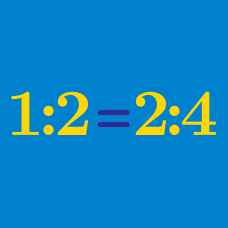Basic Mathematics

Finding Unknown Ratios

The ratio 2 to 3 is equivalent to the ratio $12 : N$. What is the value of $N$?

For what value of $N$ do we have

$5 : 8 = 25 : N ?$

For what value of $N$ do we have

$2 : 7 = 6 : N ?$

If $x$ satisfies both the proportional expression $(x-5):(x+4)=2:3$ and the linear equation $\frac{1}{3}(x-2)=\frac{3-x}{4}-(3-a)$, what is the value of the constant $a$?

For what value of $N$ do we have

$10 : 16 = 40 : N ?$

×